# Microstrip Composite Bandpass Filters for Ultra-Wideband (UWB) Wireless Communications

This example shows how to design and analyze a Low Pass Filter(LPF), High Pass Filter(HPF), and cascade responses of the LPF and HPF as well as two variations of a composite filter as shown in the paper.

The following steps are needed to create a filter:

1) Create the Geometry of the filter using RF PCB Shapes. Create a rectangle for the ground plane.

2) Use the `pcbComponent` and build the PCB stack. Set the `BoardShape` property of pcbComponent which will set the shape of the dielectric substrate.

3) Assign the filter shape, dielectric, and ground plane to the Layers property of pcbComponent.

4) Set the `FeedLocations` and `FeedDiameter`. If the design has Vias then set the `ViaLocations` and `ViaDiameter`.

5) Analyze the structure

### Create Variables

Define the variables required to create the geometry for all the filter designs

```PortLineL = 3e-3; PortLineW = 1.6e-3;```

### Low Pass Filter

Create the variables and assign the values given in the paper. Use the `traceRectangular` shape to create all the rectangles as shown in the figure and join them to create the filter structure. Visualize the structure using the `show` function.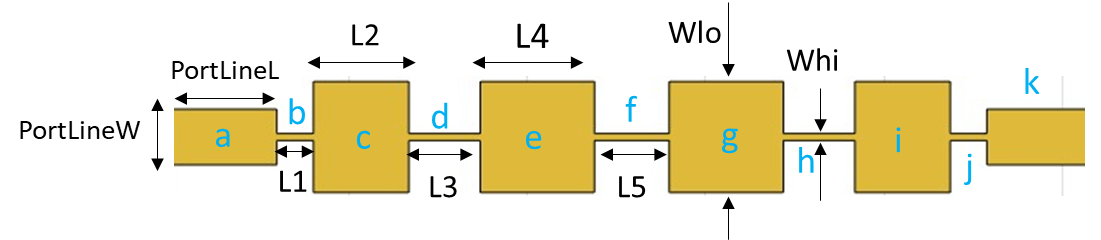```Whi = 0.2e-3; Wlo = 3.2e-3; L1 = 1.02e-3; L2 = 2.67e-3; L3 = 2e-3; L4 = 3.2e-3; L5 = 2.09e-3; a = traceRectangular('Length',PortLineL,'Width',PortLineW,'Center',[PortLineL/2,0]); b = traceRectangular('Length',L1,'Width',Whi,'Center',[PortLineL+L1/2,0]); c = traceRectangular('Length',L2,'Width',Wlo,'Center',[PortLineL+L1+L2/2,0]); d = traceRectangular('Length',L3,'Width',Whi,'Center',[PortLineL+L1+L2+L3/2,0]); e = traceRectangular('Length',L4,'Width',Wlo,'Center',[PortLineL+L1+L2+L3+L4/2,0]); f = traceRectangular('Length',L5,'Width',Whi,'Center',[PortLineL+L1+L2+L3+L4+L5/2,0]); g = traceRectangular('Length',L4,'Width',Wlo,'Center',[PortLineL+L1+L2+L3+L4+L5+L4/2,0]); h = traceRectangular('Length',L3,'Width',Whi,'Center',[PortLineL+L1+L2+L3+L4+L5+L4+L3/2,0]); i = traceRectangular('Length',L2,'Width',Wlo,'Center',[PortLineL+L1+L2+L3+L4+L5+L4+L3+L2/2,0]); j = traceRectangular('Length',L1,'Width',Whi,'Center',[PortLineL+L1+L2+L3+L4+L5+L4+L3+L2+L1/2,0]); k = traceRectangular('Length',PortLineL,'Width',PortLineW,'Center',[PortLineL+L1+L2+L3+L4+L5+L4+L3+L2+L1+PortLineL/2,0]); filt1 = a+b+c+d+e+f+g+h+i+j+k; figure,show(filt1);```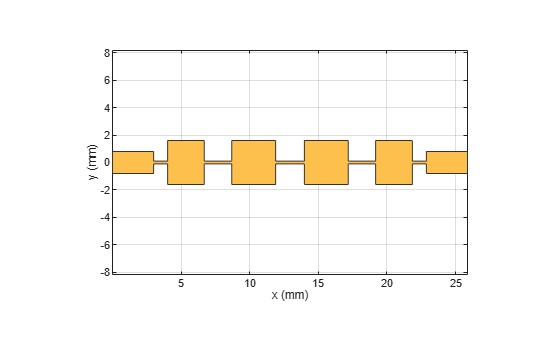Create the PCB Stack of the filter using the `pcbComponent `object. Assign the filter shape, dielectric, and groundplane to the `Layers` property. Set the `BoardThickness` to 0.508 mm and assign the `BoardShape` to the ground plane. Set the `FeedDiameter` and `FeedLocations`. Visualize the PCB.

```LPF = pcbComponent; d = dielectric('Teflon'); d.EpsilonR = 2.2; d.Thickness = 0.508e-3; GPL1 = PortLineL+L1+L2+L3+L4+L5+L4+L3+L2+L1+PortLineL; GPW = 20e-3; gnd = traceRectangular('Length',GPL1,'Width',GPW,'Center',[GPL1/2,0]); LPF.BoardThickness = 0.508e-3; LPF.Layers = {filt1,d,gnd}; LPF.BoardShape = gnd; LPF.FeedDiameter = PortLineW/2; LPF.FeedLocations = [0,0,1,3;GPL1,0,1,3]; figure,show(LPF); ```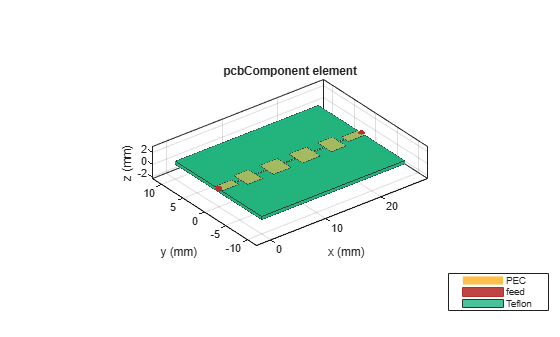Use `sparameters` function to calculate the S-Parameters of the filter and plot them using the `rfplot` function.

```spar1 = sparameters(LPF,linspace(0.1e9,16e9,51)); figure; rfplot(spar1);```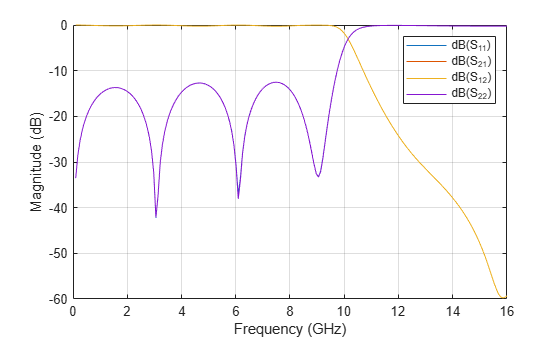### High Pass Filter

Create the variables and assign the values given in the paper. Use the `traceRectangular` shape to create all the rectangles as shown in the figure and join them to create the filter structure. Visualize the structure using the `show` function.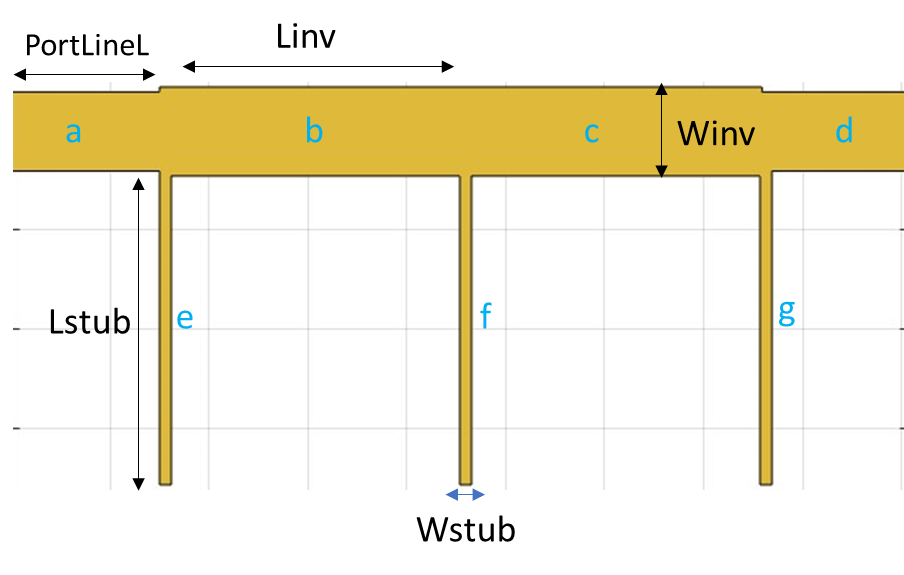```Lstub = 6.35e-3; Wstub = 0.238e-3```
```Wstub = 2.3800e-04 ```
```Linv = 6.07e-3; Winv = 1.8e-3; a = traceRectangular('Length',PortLineL,'Width',PortLineW,'Center',[PortLineL/2,0]); b = traceRectangular('Length',Linv,'Width',Winv,'Center',[PortLineL+Linv/2,0]); c = traceRectangular('Length',Linv+0.04e-3,'Width',Winv,'Center',[PortLineL+Linv+Linv/2+0.04e-3/2,0]); d = traceRectangular('Length',PortLineL+0.1e-3,'Width',PortLineW,'Center',[PortLineL+Linv+Linv+PortLineL/2-0.1e-3/2,0]); e = traceRectangular('Length',Wstub,'Width',Lstub,'Center',[PortLineL+Wstub/2,-Lstub/2-PortLineW/2]); f = traceRectangular('Length',Wstub,'Width',Lstub,'Center',[PortLineL+Linv+Wstub/2,-Lstub/2-PortLineW/2]); g = traceRectangular('Length',Wstub,'Width',Lstub,'Center',[PortLineL+Linv+Linv+Wstub/2,-Lstub/2-PortLineW/2]); filt2 = a+b+c+d+e+f+g; figure; show(filt2);```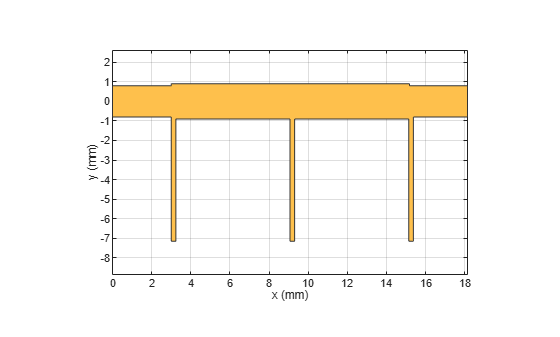Create the PCB Stack of the filter using the `pcbComponent`. Assign the filter shape, dielectric, and groundplane to the `Layers` property. Set the `BoardThickness` to 0.508 mm and assign the `BoardShape` to the ground plane. Set the `FeedDiameter` and `FeedLocations. `Visualize the PCB.

```HPF = pcbComponent; d = dielectric('Teflon'); d.EpsilonR = 2.2; d.Thickness = 0.508e-3; GPL2 = PortLineL+Linv+Linv+PortLineL; GPW = 20e-3; gnd = traceRectangular('Length',GPL2,'Width',GPW,'Center',[GPL2/2,0]); HPF.BoardThickness = 0.508e-3; HPF.Layers = {filt2,d,gnd}; HPF.BoardShape = gnd; HPF.FeedDiameter = PortLineW/2; HPF.FeedLocations = [0,0,1,3;GPL2,0,1,3]; HPF.ViaLocations = [PortLineL+Wstub/2,-PortLineW/2-Lstub+0.2e-3,1,3;PortLineL+Linv+Wstub/2,-PortLineW/2-Lstub+0.2e-3,1,3;PortLineL+Linv+Linv+Wstub/2,-PortLineW/2-Lstub+0.2e-3,1,3]; HPF.ViaDiameter = Wstub/2; figure; show(HPF);```Use `sparameters` function to calculate the S-Parameters of the filter and plot them using the `rfplot` function.

```spar2 = sparameters(HPF,linspace(0.1e9,20e9,41)); figure; rfplot(spar2);```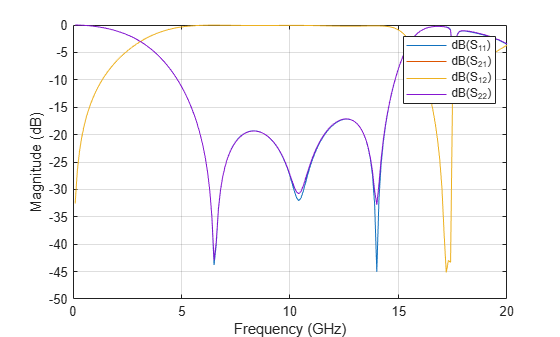Cascade the LPF and HPF created above and create a ground plane so that it supports the cascaded shape.

```filt12 = translate(copy(filt1),[-GPL1,0,0]); filtcasc = filt12+filt2; figure,show(filtcasc);```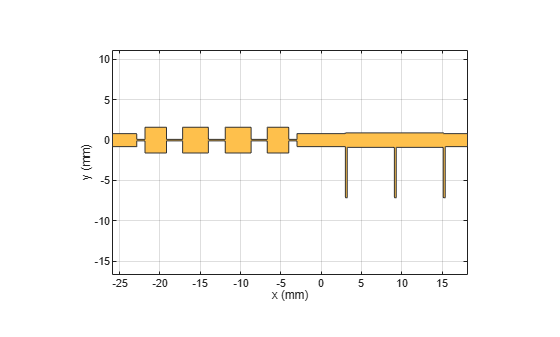Create the PCB Stack of the filter using the `pcbComponent`. Create the groundplane using the `traceRectangular `shape`. `Assign the filter shape, dielectric, and groundplane to the `Layers` property. Set the `BoardThickness` to 0.508 mm and assign the `BoardShape` to the ground plane. Set the `FeedDiameter` and `FeedLocations. `Visualize the PCB.

```casc = pcbComponent; d = dielectric('Teflon'); d.EpsilonR = 2.2; d.Thickness = 0.508e-3; GPL = GPL1+GPL2; GPW = 20e-3; gnd = traceRectangular('Length',GPL,'Width',GPW,'Center',[(-GPL1+GPL2)/2,0]); GPL3 = PortLineL+Linv+Linv+PortLineL; casc.BoardThickness = 0.508e-3; casc.Layers = {filtcasc,d,gnd}; casc.BoardShape = gnd; casc.FeedDiameter = PortLineW/2; casc.FeedLocations = [-GPL1,0,1,3;GPL3,0,1,3]; casc.ViaLocations = [PortLineL+Wstub/2,-PortLineW/2-Lstub+0.2e-3,1,3;PortLineL+Linv+Wstub/2,-PortLineW/2-Lstub+0.2e-3,1,3;PortLineL+Linv+Linv+Wstub/2,-PortLineW/2-Lstub+0.2e-3,1,3]; casc.ViaDiameter = Wstub/2; figure; show(casc);```Use the `sparameters` function to calculate the S-Parameters and plot the parameters using the `rfplot` function

```spar3 = sparameters(casc,linspace(0.1e9,14e9,31)); figure; rfplot(spar3);```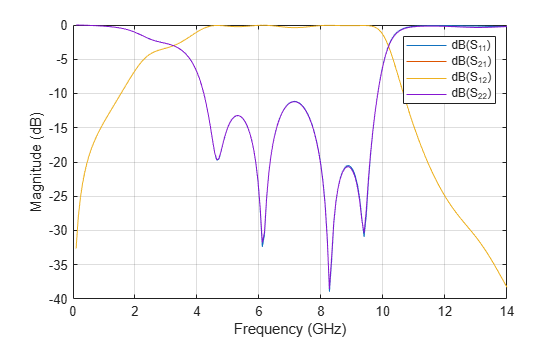### Composite Filter Type 1

Create the variables and assign the values given in the paper. Use the `traceRectangular` shape to create all the rectangles as shown in the figure and join them to create the filter structure. Visualize the structure using the `show` function.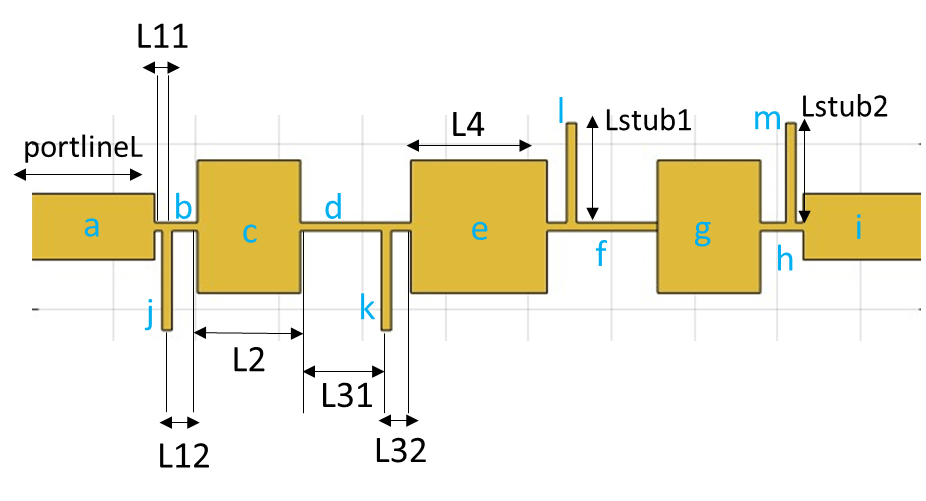```L11 = 0.3e-3; L12 = 0.73e-3; L2 = 2.48e-3; L31 = 2.07e-3; L32 = 0.59e-3; L4 = 3.28e-3; Lstub1 = 2.4e-3; Lstub2 = 2.4e-3; a = traceRectangular('Length',PortLineL,'Width',PortLineW,'Center',[PortLineL/2,0]); b = traceRectangular('Length',L11+L12,'Width',Whi,'Center',[PortLineL+(L11+L12)/2,0]); c = traceRectangular('Length',L2,'Width',Wlo,'Center',[PortLineL+L11+L12+L2/2,0]); d = traceRectangular('Length',L31+L32,'Width',Whi,'Center',[PortLineL+L11+L12+L2+(L31+L32)/2,0]); e = traceRectangular('Length',L4,'Width',Wlo,'Center',[PortLineL+L11+L12+L2+(L31+L32)+L4/2,0]); f = traceRectangular('Length',L31+L32,'Width',Whi,'Center',[PortLineL+L11+L12+L2+(L31+L32)+L4+(L31+L32)/2,0]); g = traceRectangular('Length',L2,'Width',Wlo,'Center',[PortLineL+L11+L12+L2+(L31+L32)+L4+(L31+L32)+L2/2,0]); h = traceRectangular('Length',L11+L12,'Width',Whi,'Center',[PortLineL+L11+L12+L2+(L31+L32)+L4+(L31+L32)+L2+(L11+L12)/2,0]); i = traceRectangular('Length',PortLineL,'Width',PortLineW,'Center',[PortLineL+L11+L12+L2+(L31+L32)+L4+(L31+L32)+L2+(L11+L12)+PortLineL/2,0]); j = traceRectangular('Length',Wstub,'Width',Lstub1,'Center',[PortLineL+L11,-Lstub1/2-Whi/2]); k = traceRectangular('Length',Wstub,'Width',Lstub1,'Center',[PortLineL+L11+L12+L2+L31,-Lstub1/2-Whi/2]); l = traceRectangular('Length',Wstub,'Width',Lstub1,'Center',[PortLineL+L11+L12+L2+L31+L32+L4+L32,Lstub1/2+Whi/2]); m = traceRectangular('Length',Wstub,'Width',Lstub1,'Center',[PortLineL+L11+L12+L2+L31+L32+L4+L32+L31+L2+L12,Lstub1/2+Whi/2]); compfiltShape1 = a+b+c+d+e+f+g+h+i+j+k+l+m; figure; show(compfiltShape1);```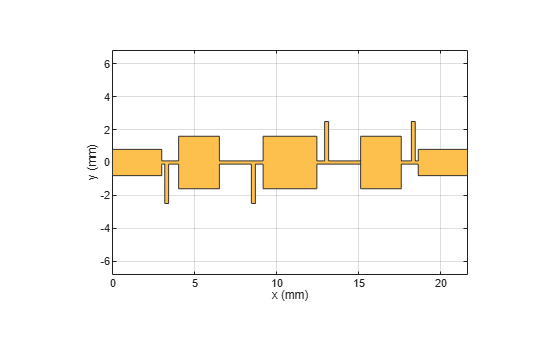Create the PCB Stack of the filter using the `pcbComponent`. Assign the filter shape, dielectric, and groundplane to the `Layers` property. Set the `BoardThickness` to 0.508 mm and assign the `BoardShape` to the ground plane. Set the `FeedDiameter` and `FeedLocations. `Visualize the PCB.

```compfilt1 = pcbComponent; d = dielectric('Teflon'); d.EpsilonR = 2.2; d.Thickness = 0.508e-3; GPL2 = PortLineL+2*(L11+L12)+2*(L31+L32)+2*L2+L4+PortLineL; GPW = 20e-3; gnd = traceRectangular('Length',GPL2,'Width',GPW/2,'Center',[(GPL2)/2,0]); compfilt1.BoardThickness = 0.508e-3; compfilt1.Layers = {compfiltShape1,d,gnd}; compfilt1.BoardShape = gnd; compfilt1.FeedDiameter = PortLineW/2; compfilt1.FeedLocations = [0,0,1,3;GPL2,0,1,3]; compfilt1.ViaLocations = [PortLineL+L11,-Whi/2-Lstub1+0.1e-3,1,3;PortLineL+L11+L12+L2+L31,-Whi/2-Lstub1+0.1e-3,1,3;PortLineL+L11+L12+L2+L31+L32+L4+L32,Whi/2+Lstub1-0.1e-3,1,3;PortLineL+L11+L12+L2+L31+L32+L4+L32+L31+L2+L12,Whi/2+Lstub1-0.1e-3,1,3]; compfilt1.ViaDiameter = Wstub/2; figure; show(compfilt1);```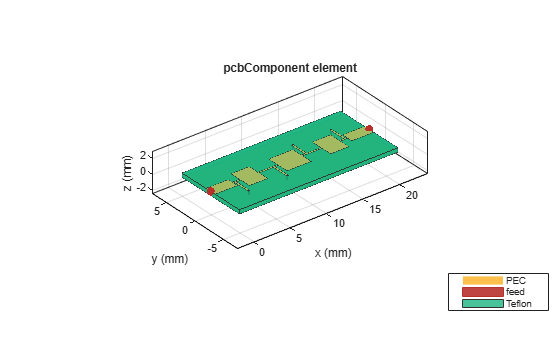Use the `mesh` function to generate a manual mesh with a `MaxEdgeLength` as 1 mm.

```figure; mesh(compfilt1,'MaxEdgeLength',1.2e-3);```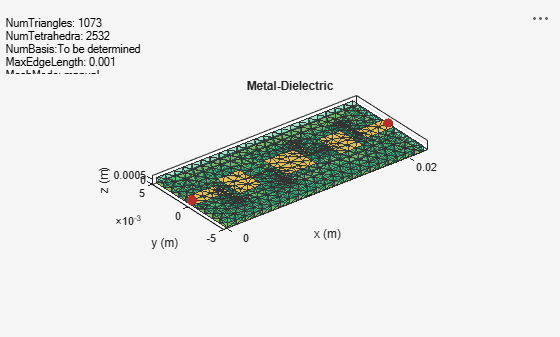Use `sparameters` function to calculate the S-Parameters of the filter and plot them using the `rfplot` function.

```spar4 = sparameters(compfilt1,linspace(0.1e9,14e9,31)); figure; rfplot(spar4);```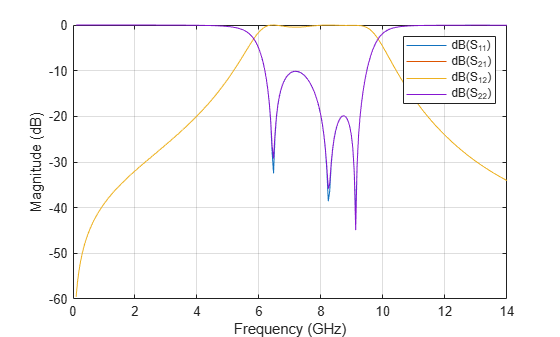### Composite Filter Type 2

Create the variables and assign the values given in the paper. Use the `traceRectangular` shape to create all the rectangles as shown in the figure and join them to create the filter structure. Visualize the structure using the `show` function.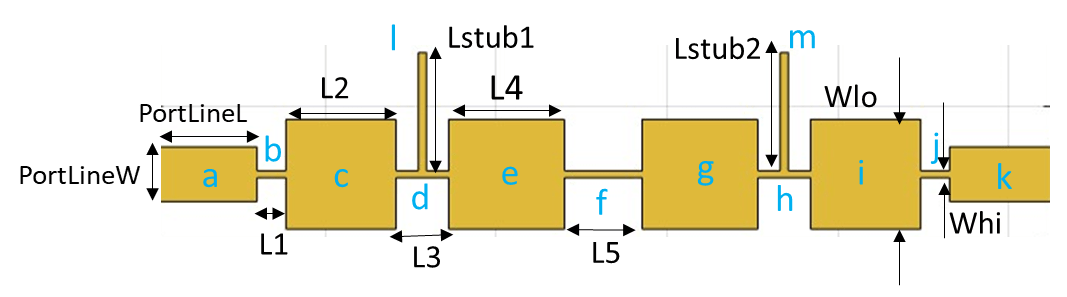```Whi = 0.2e-3; Wlo = 3.2e-3; L1 = 0.85e-3; L2 = 3.22e-3; L3 = 1.54e-3; L4 = 3.39e-3; L5 = 2.27e-3; Lstub1 = 3.45e-3; a = traceRectangular('Length',PortLineL,'Width',PortLineW,'Center',[PortLineL/2,0]); b = traceRectangular('Length',L1,'Width',Whi,'Center',[PortLineL+L1/2,0]); c = traceRectangular('Length',L2,'Width',Wlo,'Center',[PortLineL+L1+L2/2,0]); l = traceRectangular('Length',Wstub,'Width',Lstub1,'Center',[PortLineL+L1+L2+L3/2,Lstub1/2+Whi/2]); d = traceRectangular('Length',L3,'Width',Whi,'Center',[PortLineL+L1+L2+L3/2,0]); e = traceRectangular('Length',L4,'Width',Wlo,'Center',[PortLineL+L1+L2+L3+L4/2,0]); f = traceRectangular('Length',L5,'Width',Whi,'Center',[PortLineL+L1+L2+L3+L4+L5/2,0]); g = traceRectangular('Length',L4,'Width',Wlo,'Center',[PortLineL+L1+L2+L3+L4+L5+L4/2,0]); m = traceRectangular('Length',Wstub,'Width',Lstub1,'Center',[PortLineL+L1+L2+L3+L4+L5+L4+L3/2,Lstub1/2+Whi/2]); h = traceRectangular('Length',L3,'Width',Whi,'Center',[PortLineL+L1+L2+L3+L4+L5+L4+L3/2,0]); i = traceRectangular('Length',L2,'Width',Wlo,'Center',[PortLineL+L1+L2+L3+L4+L5+L4+L3+L2/2,0]); j = traceRectangular('Length',L1,'Width',Whi,'Center',[PortLineL+L1+L2+L3+L4+L5+L4+L3+L2+L1/2,0]); k = traceRectangular('Length',PortLineL,'Width',PortLineW,'Center',[PortLineL+L1+L2+L3+L4+L5+L4+L3+L2+L1+PortLineL/2,0]); compfiltShape2 = a+b+c+d+e+f+g+h+i+j+k+l+m; figure; show(compfiltShape2);```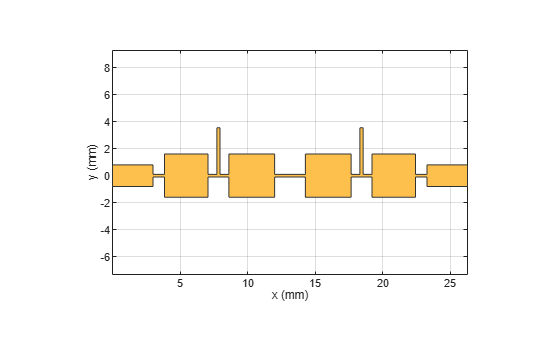Create the PCB Stack of the filter using the `pcbComponent`. Assign the filter shape, dielectric, and groundplane to the `Layers` property. Set the `BoardThickness` to 0.508 mm and assign the `BoardShape` to the ground plane. Set the `FeedDiameter` and `FeedLocations.` Visualize the PCB.

```compfilt2 = pcbComponent; d = dielectric('Teflon'); d.EpsilonR = 2.2; d.Thickness = 0.508e-3; GPL1 = PortLineL+L1+L2+L3+L4+L5+L4+L3+L2+L1+PortLineL; GPW = 20e-3; gnd = traceRectangular('Length',GPL1,'Width',GPW/2,'Center',[GPL1/2,0]); compfilt2.BoardThickness = 0.508e-3; compfilt2.Layers = {compfiltShape2,d,gnd}; compfilt2.BoardShape = gnd; compfilt2.FeedDiameter = PortLineW/2; compfilt2.FeedLocations = [0,0,1,3;GPL1,0,1,3]; compfilt2.ViaLocations = [PortLineL+L1+L2+L3/2,Lstub1+Whi/2-0.2e-3,1,3;PortLineL+L1+L2+L3+L4+L5+L4+L3/2,Lstub1+Whi/2-0.2e-3,1,3]; compfilt2.ViaDiameter = Wstub/2; figure; show(compfilt2);```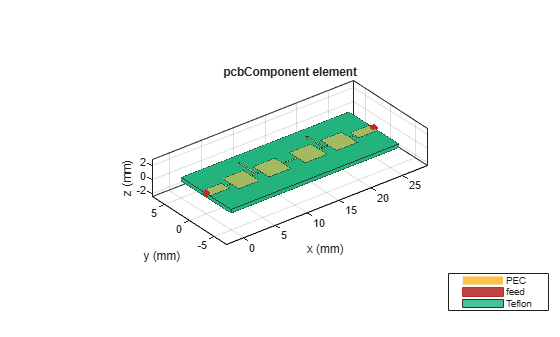Use the `mesh` function to generate a manual mesh with a `MaxEdgeLength` as 1.5 mm.

```figure; mesh(compfilt2,'MaxEdgeLength',1.5e-3);```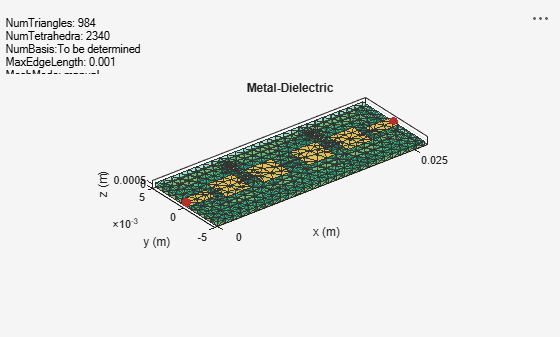Use `sparameters` function to calculate the S-Parameters of the filter and plot them using the `rfplot` function.

```spar5 = sparameters(compfilt2,linspace(0.5e9,14e9,41)); figure; rfplot(spar5);```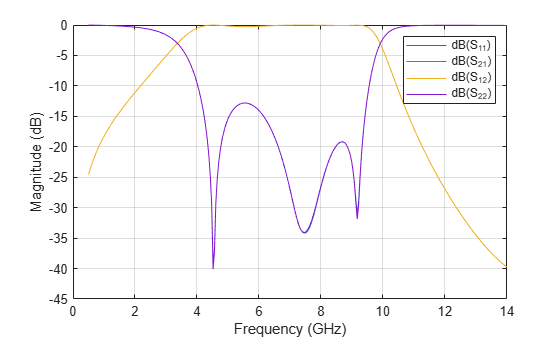### References

1) Ching-Luh Hsu, Fu-Chieh Hsu and Jen-Tsai Kuo, Microstrip Bandpass Filters for Ultra-Wideband (UWB) Wireless Communications.